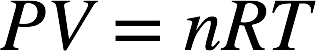# Problem: Consider the following reaction:4Al(s) + 3O2(g) → 2Al2O3(s)It takes 2.00 L of pure oxygen gas at STP to react completely with a certain sample of aluminum. What is the mass of aluminum reacted?

🤓 Based on our data, we think this question is relevant for Professor Paesani's class at UCSD.

###### FREE Expert Solution

The ideal gas law is as follows:At conditions of standard temperature and pressure (STP), temperature = 273.15 K and pressure = 1 atm. The volume of the O2(g) is 2.00 L. From these values, we can calculate the moles of O2(g):###### Problem Details

Consider the following reaction:

4Al(s) + 3O2(g) → 2Al2O3(s)

It takes 2.00 L of pure oxygen gas at STP to react completely with a certain sample of aluminum. What is the mass of aluminum reacted?